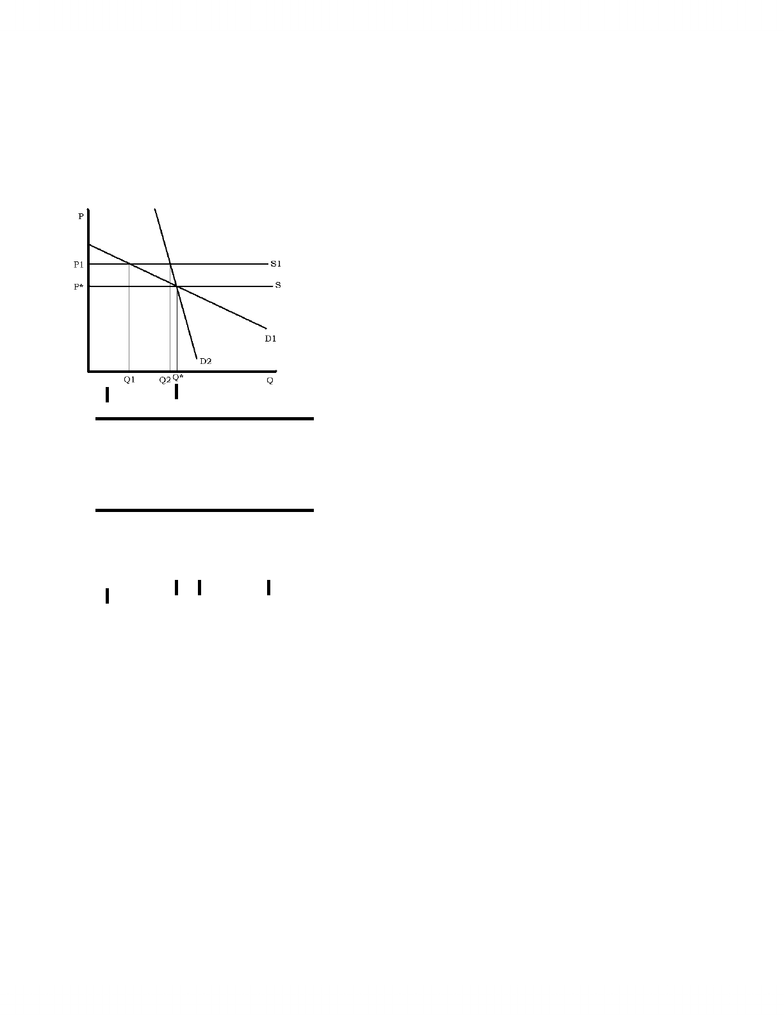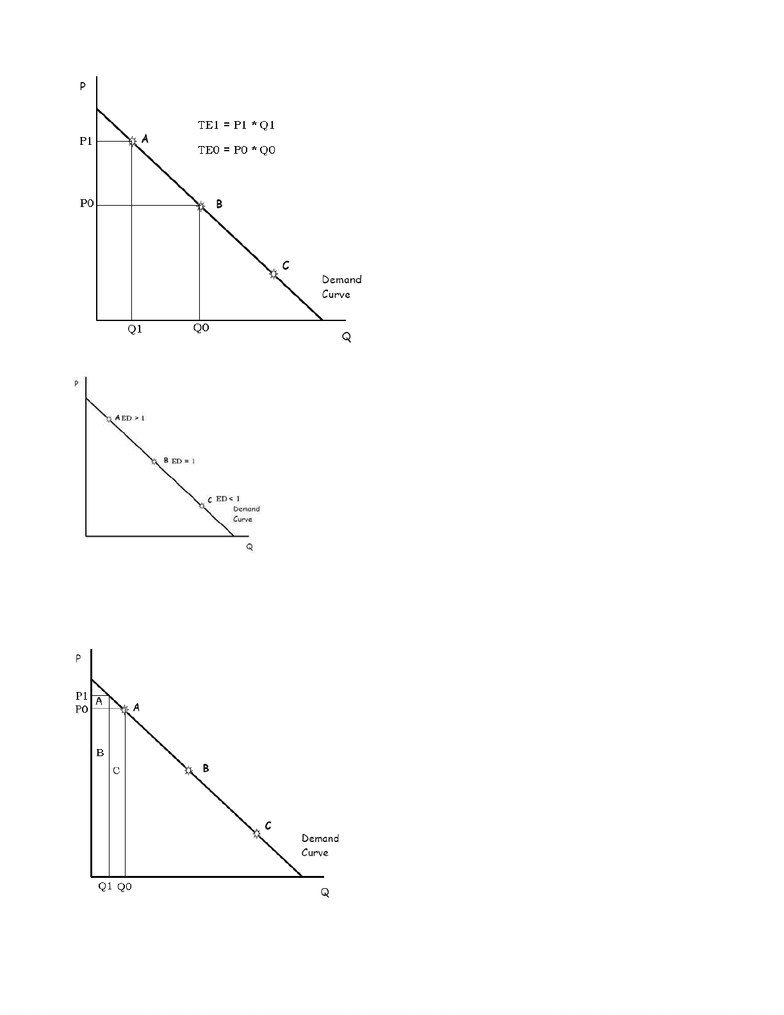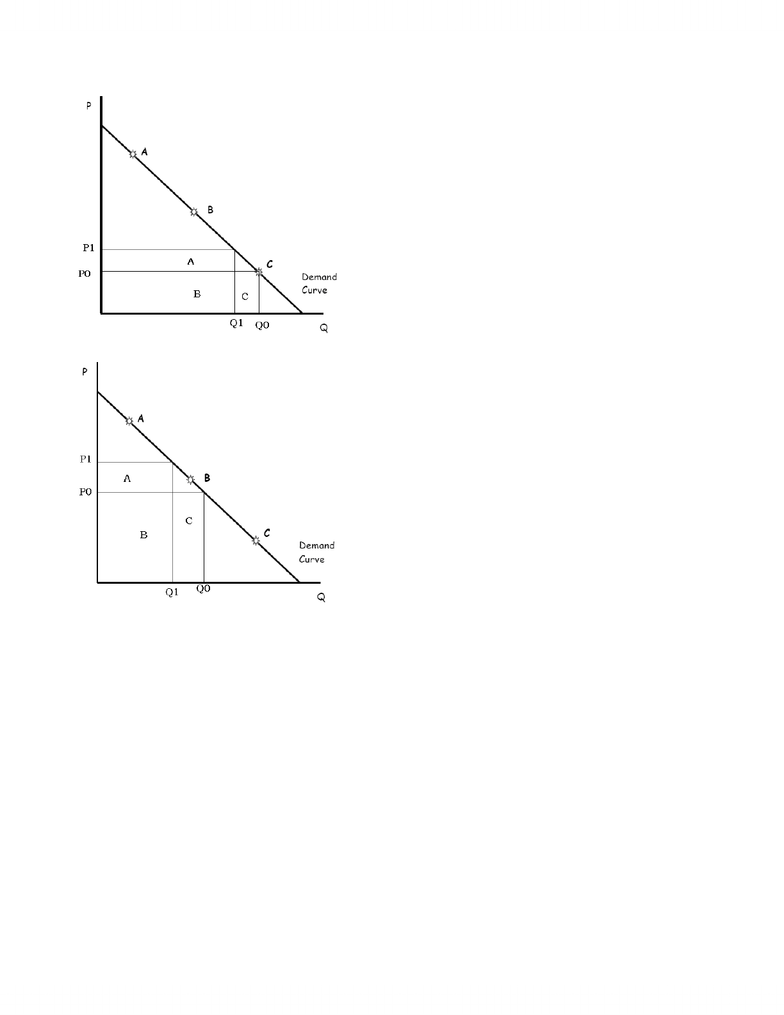# MGEA02H3 Lecture Notes - Lecture 4: Lux, Plywood

39 views9 pagesWeek 4 – Elasticity, Tax Incidence and Tax Burden
A key characteristic of demand and supply curves! – Elasticity
Elasticity = sensitivity or responsiveness
Changes in one variable as another variable changes
How sensitive is the quantity demanded to changes in the price of the product? Answer is given by elasticity of demand.
How sensitive is the quantity of tomatoes supplied by businesses to changes in the price of tomatoes in Ontario? Answer is given by the
elasticity of supply of tomatoes in Ontario.
Elasticity of Demand
ED = dQ/dP x P/Q = (dQ / Q) / (dP / P)
ED = percentage change in quantity demanded
percentage change in price
….how consumers respond to a change in market price
Elasticity of Supply
ES = dQ/dP x P/Q
ES = percentage change in quantity supplied
percentage change in price
…how producers respond to a change in market price
If demand is P = 100 – Q, what is the elasticity of demand at Q = 80?
At Q = 80, P = \$20
ED = dQ/dP x P/Q = 1/(-1) x 20/80 = ¼
What does it mean to say that the elasticity of demand at this point is ¼?
It means that if P = \$20 and the price changes by 4%, we expect that the quantity demanded will change by about 1%.
Terminology:
Demand is elastic if ED > 1
Demand is inelastic if ED < 1
Demand is unit(ary) elastic if ED = 1
Think about relation between consumers’ total expenditure on a product and the price that firms charge to customers. (It depends on the
elasticity of demand).
Consider dTE/dP. TE = P x Q
Both are functions of P
Use product rule.
dTE/dP = (dP/dP x Q) + (dQ/dP x P) = Q + dQ/dP x P
Multiply by Q/Q = Q(Q/Q + dQ/dP x P/Q)
So dTE/dP = Q(1 – ED)
So, if ED > 1, dTE/dP < 0
And if ED < 1, dTE/dP > 0
We can see this on a graph of the demand curve as well….
www.notesolution.com
Unlock document

This preview shows pages 1-3 of the document.
Unlock all 9 pages and 3 million more documents.Strange, but true, fact
Elasticity is different at every different point along a linear demand curve
Thinking about different goods, some have more elastic demands; others have less elastic demands.
What affects elasticity of demand?
Availability of close substitutes is key
Also amount spent on this good by the consumer
How are elasticity of demand and changes in total expenditure by consumers (i.e., the revenue of the producer) related?
See it on a graph (when demand is elastic):
www.notesolution.com
Unlock document

This preview shows pages 1-3 of the document.
Unlock all 9 pages and 3 million more documents.When demand is inelastic
When demand is unit elastic
When demand is elastic, a fall in price will raise total expenditure
When demand is elastic, a rise in price will lower total expenditure
When demand is inelastic, a fall in price will lower total expenditure
When demand is inelastic, a rise in price will raise total expenditure
When demand is unit elastic, a fall or a rise in price will have (approximately) no effect on total expenditure
Elasticity and Taxation
An illustration of the use ofelasticity
Think about the tax on one particular product (not a general tax on many products)
– e.g., gasoline, liquor, cigarettes
This is called an “excise” tax
Could be a “flat-rate” tax
e.g., \$5 per bottle of liquor, \$0.30 per litre of gasoline, \$10 per carton of cigarettes
e.g., 10% of the price on a bottle of liquor, 25% on a litre of gasoline, 30% on a carton of cigarettes
To keep it simple, we look only at flat-rate excise tax.
www.notesolution.com
Unlock document

This preview shows pages 1-3 of the document.
Unlock all 9 pages and 3 million more documents.

## Document Summary

Week 4 elasticity, tax incidence and tax burden. A key characteristic of demand and supply curves! Changes in one variable as another variable changes. Answer is given by the elasticity of supply of tomatoes in ontario. Ed = dq/dp x p/q = (dq / q) / (dp / p) Ed = percentage change in quantity demanded percentage change in price. how consumers respond to a change in market price. Es = percentage change in quantity supplied percentage change in price. How producers respond to a change in market price. Ed = dq/dp x p/q = 1/(-1) x 20/80 = . It means that if p = and the price changes by 4%, we expect that the quantity demanded will change by about 1%. (why about ?) Demand is unit(ary) elastic if ed = 1. Think about relation between consumers" total expenditure on a product and the price that firms charge to customers. (it depends on the elasticity of demand).

## Get access

\$10 USD/m
Billed \$120 USD annuallyHomework Help
Study Guides
Textbook Solutions
Class Notes
Textbook Notes
Booster Class